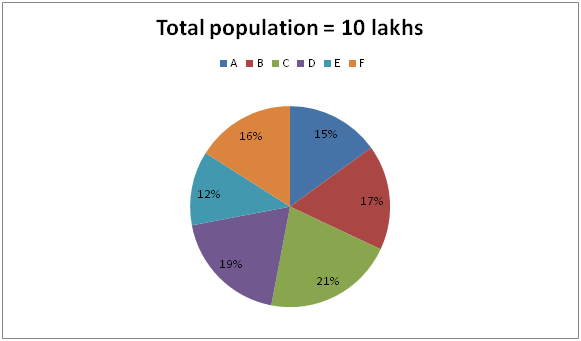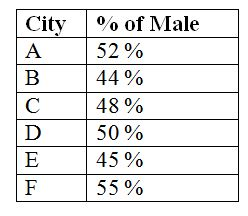# SBI PO Prelims Quantitative Aptitude Questions 2019 (Day-50)

Dear Aspirants, Our IBPS Guide team is providing new series of Quantitative Aptitude Questions for SBI PO 2019 so the aspirants can practice it on a daily basis. These questions are framed by our skilled experts after understanding your needs thoroughly. Aspirants can practice these new series questions daily to familiarize with the exact exam pattern and make your preparation effective.

[WpProQuiz 6384]

### Click Here for SBI PO Pre 2019 High-Quality Mocks Exactly on SBI Standard

Directions (Q. 1 – 5): What value should come in place of (?) in the following questions?

1) 1/10 + 3/20 + 4/30 =? – 2 3/4

a) 3 2/15

b) 4 7/10

c) 2 8/15

d) 5 9/10

e) None of these

2) 24 % of 150 + (4/9) of 270 – 45 ÷ 9 × 11 = ?

a) 158

b) 217

c) 272

d) 101

e) None of these

3) 122 + 3.5 % of 1200 – 93 = ? – 845

a) 418

b) 302

c) 356

d) 474

e) None of these

4) 5 9/16 + 34/16 – (17/12) × 3 = ? – 4 7/8

a) 7 5/8

b) 9 11/16

c) 8 5/16

d) 10 3/8

e) None of these

5) (2/7) of (3/8) of (14/21) of 448 = ?

a) 32

b) 45

c) 26

d) 51

e) None of these

Directions (Q. 6 – 10):  Study the following information carefully and answer the questions given below:

The following pie chart shows the percentage distribution of total population (Male and Female) of 6 different cities in a certain state.The following table shows the percentage of male among them.6) Find the difference between the total female population city A to that of total male population of city E?

a) 24000

b) 18000

c) 32000

d) 28000

e) None of these

7) Find the total literate population of city B to that of total male population of city F, if the ratio between the total literate to that of illiterate population in city B is 10 : 7?

a) 156000

b) 172000

c) 164000

d) 188000

e) None of these

8) Find the average male population in city C, D and F together?

a) 94600

b) 102800

c) 118400

d) 125200

e) None of these

9) Total female population in city C is approximately what percentage of total male population in city F?

a) 136 %

b) 115 %

c) 124 %

d) 164 %

e) 175 %

10) Find the ratio between the total illiterate population in city D to that of total literate population in city E, if the ratio between the total literate to that of illiterate population in city D is 11 : 8 and the percentage of total illiterate population in city E is 32 %?

a) 50 : 51

b) 37 : 39

c) 5 : 7

d) 11 : 12

e) None of these

1/10 + 3/20 + 4/30 + 11/4 = X

x = (12 + 18 + 16 + 330)/120

x = 376/120 = 3 2/15

(24/100) * 150 + (4/9) * 270 – (45/9) * 11 =  x

x = 36 + 120 – 55 = 101

144 + (3.5/100) * 1200 – 729 + 845 = x

x = 144 + 42 – 729 + 845

x = 302

5 9/16 + 2 1/8 – (17/12) × 3 = x – 4 7/8

5 9/16 + 2 1/8 – 4 ¼ + 4 7/8 = x

x = (5 + 2 – 4 + 4) [(9 + 2 – 4 + 14)/16]

x = 7 (21/16) = 8 5/16

(2/7) * (3/8) * (14/21) * 448  =  x

x = 32

Directions (Q. 6 – 10):

The total female population city A

= > 1000000*(15/100)*(48/100) = 72000

The total male population of city E

= > 1000000*(12/100)*(45/100) = 54000

Required difference = 72000 – 54000 = 18000

The total literate population of city B

= > 1000000*(17/100)*(10/17) = 100000

The total male population of city F

= > 1000000*(16/100)*(55/100) = 88000

Required total = 100000 + 88000 = 188000

The average male population in city C, D and F together

= > [1000000*(21/100)*(48/100) + 1000000*(19/100)*(50/100) + 1000000*(16/100)*(55/100)]/3

= > (100800 + 95000 + 88000)/3

= > 283800/3 = 94600

Total female population in city C

= > 1000000 * (21/100) * (52/100)

Total male population in city F

= > 1000000 * (16/100) * (55/100)

Required % = {[10 * (21/100) * (52/100)]/[10 * (16/100) * (55/100)]} * 100

= > 124.09 % = 124 %

The total illiterate population in city D

= > 1000000 * (19/100) * (8/19)

The total literate population in city E

= > 1000000 * (12/100) * (68/100)

Required ratio = [1000000 * (19/100) * (8/19)] : [1000000 * (12/100) * (68/100)]

= > 50 : 51

Related Articles :